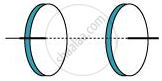Share

# A Capacitor Made of Two Circular Plates Each of Radius 12 cm, and Separated by 5.0 cm. the Capacitor is Being Charged by an External Source (Not Shown in the Figure). the Charging Current is Constant and Equal to 0.15 A. - Physics

#### Question

Figure 8.6 shows a capacitor made of two circular plates each of radius 12 cm, and separated by 5.0 cm. The capacitor is being charged by an external source (not shown in the figure). The charging current is constant and equal to 0.15 A.

(a) Calculate the capacitance and the rate of charge of potential difference between the plates.

(b) Obtain the displacement current across the plates.#### Solution

Radius of each circular plate, r = 12 cm = 0.12 m

Distance between the plates, d = 5 cm = 0.05 m

Charging current, I = 0.15 A

Permittivity of free space, epsilon_0= 8.85 × 10−12 C2 N−1 m−2

(a) Capacitance between the two plates is given by the relation,

C =(in_0pir^2)/d

Where,

A = Area of each plate =pir^2

C=(in_0pir^2)/d

=(8.85XX10^-12xxpixx(0.12)^2)/0.05

=8.0032xx10^-12F=80.032 pF

Charge on each plate, q = CV

Where,

V = Potential difference across the plates

Differentiation on both sides with respect to time (t) gives:

(dq)/(dt)=C(dV)/(dt)

But , (dq)/(dt)=current(I)

therefore (dV)/(dt)=I/C

=>(0.15)/(80.032xx10^-12)=1.87xx10^9 V/s

Therefore, the change in potential difference between the plates is 1.87 ×109 V/s.

(b) The displacement current across the plates is the same as the conduction current. Hence, the displacement current, id is 0.15 A.

(c) Yes

Kirchhoff’s first rule is valid at each plate of the capacitor provided that we take the sum of conduction and displacement for current.

Is there an error in this question or solution?